# Understanding the mathematics behind Support Vector Machines

Support Vector Machine (SVM) is one of the most powerful out-of-the-box supervised machine learning algorithms. Unlike many other machine learning algorithms such as neural networks, you don’t have to do a lot of tweaks to obtain good results with SVM. I spent quite a time reading articles, blogs, and online materials trying to get the gist of this performant algorithm and found that most of the tutorials out online are explaining SVM from a big overview, while treating the underlying mathematical background as a block box. I often still felt confused after I finished reading the whole article. What exactly is the problem SVM is trying to solve? How do we get the optimal hyperplane? How does SVM handle non-linearly separable data? Why use kernels? To fully understand the answers to these questions, we need to go under the hood and explore the mathematics behind SVMs and understand how they work.

In this blog post, I will focus on the underlying mathematical part of SVMs and this requires that you have at least some background in linear algebra and optimization theory.

## Definitions

Before we get into the SVM algorithm, let’s first talk about some definitions we need to use later.

### Length of a vector

The length of a vector x is called its norm, which is written as ||x||. The Euclidean norm formula to calculate the norm of a vector x = ($x_1, x_2, ..., x_n$) is:

$||x|| = \sqrt{x_1^2+x_2^2+...+x_n^2}$

### Direction of a vector

The direction of a vector x = ($x_1, x_2$) is written as w, and is defined as:

$w = (\frac{x_1}{||x||},\frac{x_2}{||x||})$

If we look at figure 1, we can see that $cos(\theta)=\frac{x_1}{\|x\|}$ and $cos(\alpha)=\frac{x_2}{\|x\|}$. Thus, the direction vector w can also be written as:

$w = (cos(\theta), cos(\alpha))$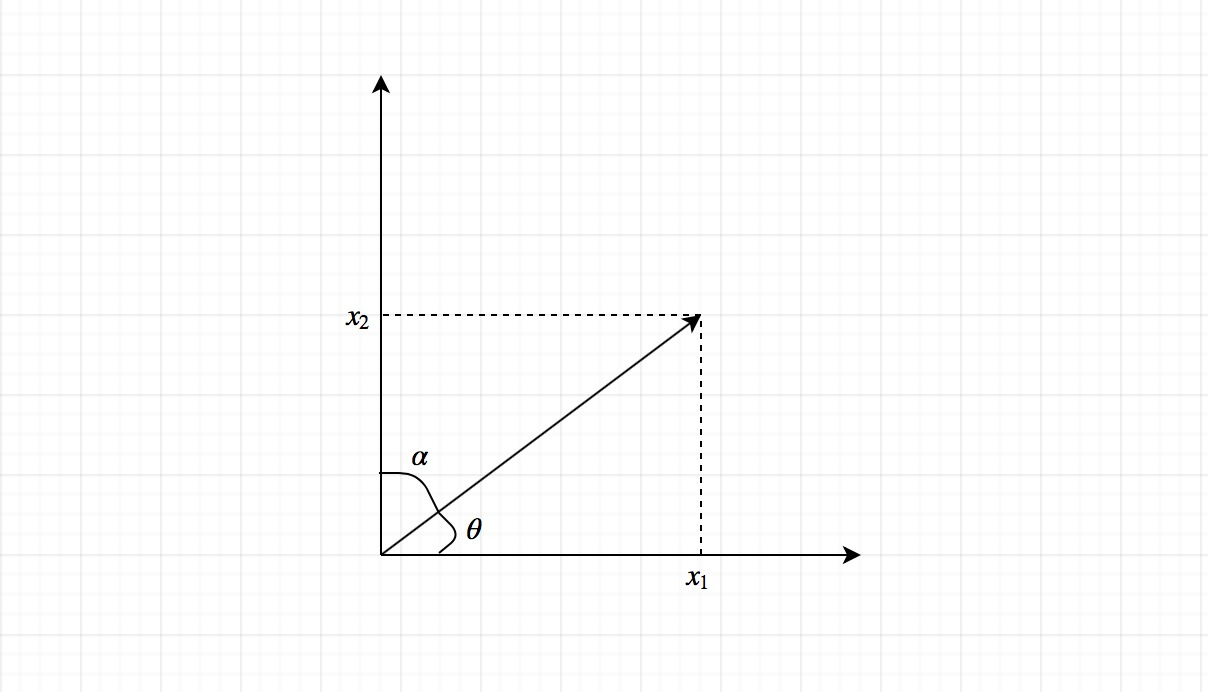It is worth noting that the norm of a direction vector is always equal to 1. Because of this, the direction vector w is also called the unit vector.

### Dot product

The dot product of two vectors returns a scalar. It gives us some insights into how the two vectors are related.

Figure 2 shows two vectors x and y and the angle $\theta$ between them. The geometric formula of dot product is defined as:

$x \cdot y = ||x||\space||y||\space cos(\theta)$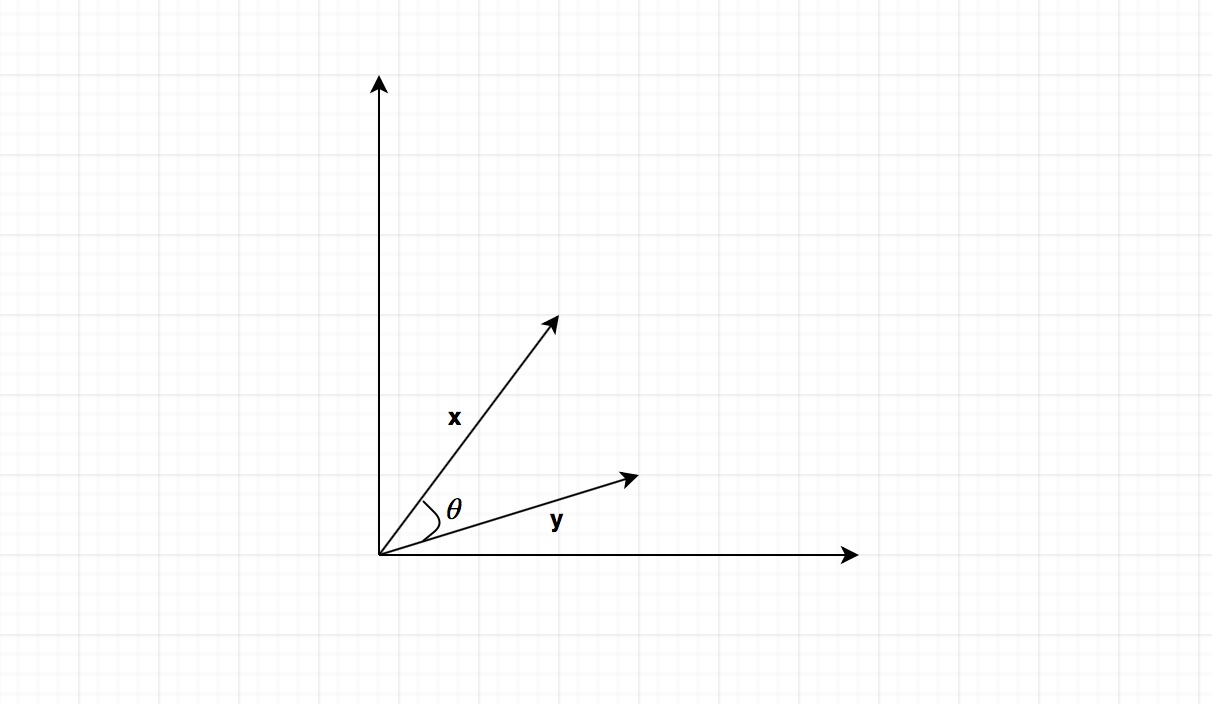By looking at figure 3, we can see $\theta=\beta-\alpha$. Then we can get:

$cos(\theta) = cos(\beta-\alpha)\\ =cos\beta cos\alpha+sin\beta sin\alpha \\ =\frac{x_1}{||x||}\frac{y_1}{||y||}+\frac{x_2}{||x||}\frac{y_2}{||y||} \\ =\frac{x_1y_1+x_2y_2}{||x||\space ||y||}$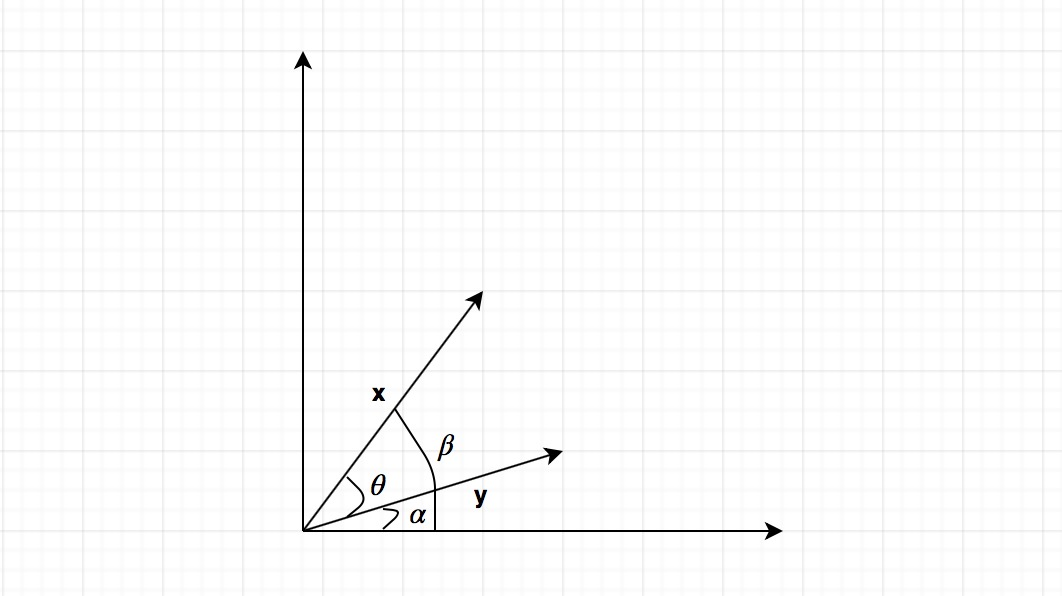We substitute this into the geometric dot product formula, we get:

$x \cdot y = ||x||\space||y||\space \frac{x_1y_1+x_2y_2}{||x||\space ||y||} = x_1y_1+x_2y_2$

This is the algebraic formula of dot product. In general, dot product can be computed as the following for two n-dimensional vectors:

$x \cdot y = \sum_{i=1}^{n}x_iy_i$

### Linear separability

Linear separability is one important concept in SVM. Although in practical cases the data might not be linearly separable, we will start from the linearly separable cases (since they are easy to understand and deal with) and then derive the non-linearly separable cases.

Figure 4 shows the two-dimensional data are separated by a line. In this case, we say the data are linearly separable. Figure 5 is an example of non-linearly separable data, which means we can not find a line to separate the two-dimensional data. Similarly, for three-dimensional data, we say the data are linearly separable if we can find a plane to separate them.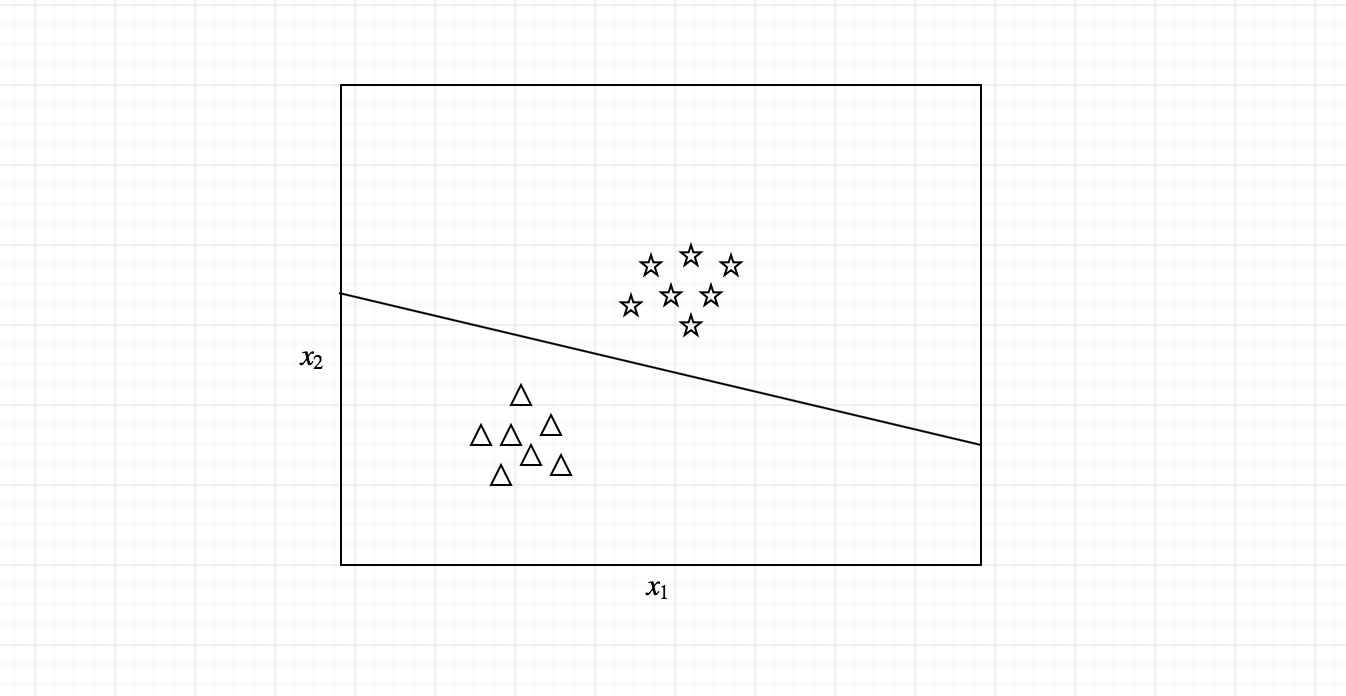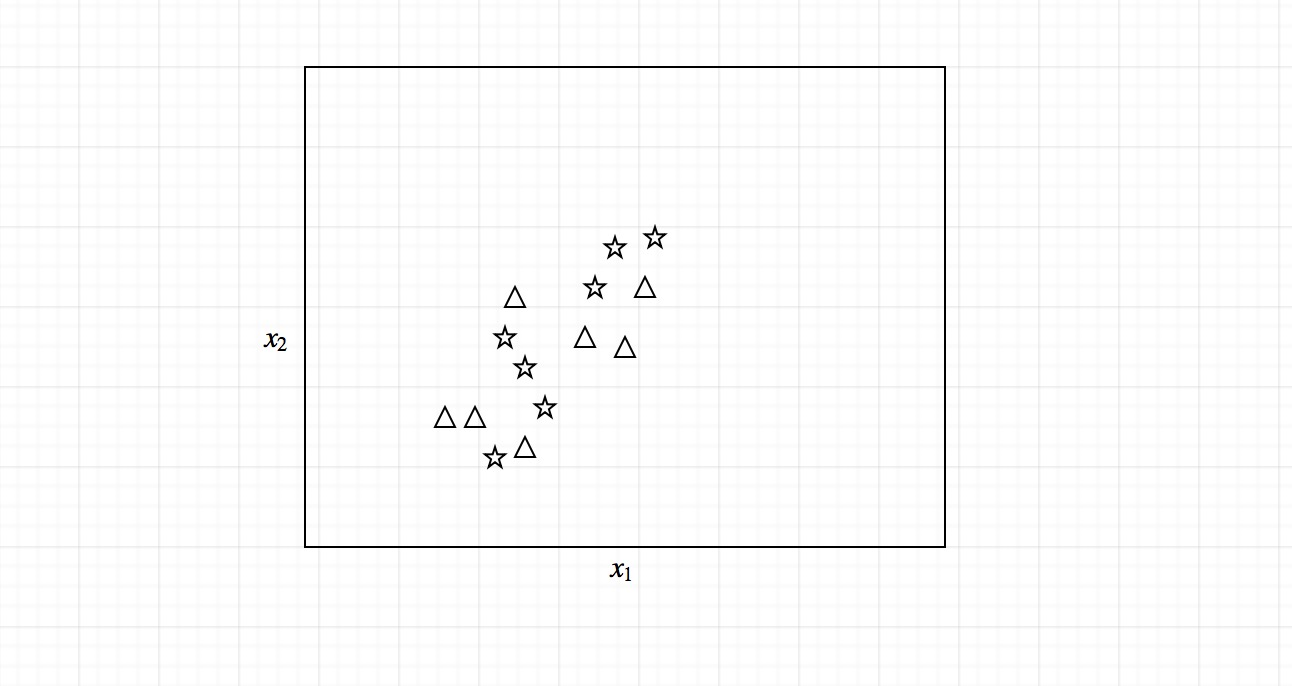### Hyperplane

Then, the question arises when there are more than three dimensions. What do we use to separate the multi-dimensional data? We use hyperplane. How could we define a hyperplane?

Let’s look at the two-dimensional case first. The two-dimensional linearly separable data can be separated by a line. The function of the line is $y=ax+b$. We rename x with $x_1$ and y with $x_2$ and we get:

$ax_1-x_2+b=0$

If we define x = $(x_1,x_2)$ and w = $(a,-1)$, we get:

$w\cdot x + b =0$

This equation is derived from two-dimensional vectors. But in fact, it also works for any number of dimensions. This is the equation of the hyperplane.

### Classifier

Once we have the hyperplane, we can then use the hyperplane to make predictions. We define the hypothesis function h as:

$h(x_i) = \begin{cases} +1 & if \space w\cdot x + b \ge 0 \\ -1 & if \space w\cdot x + b < 0 \end{cases}$

The point above or on the hyperplane will be classified as class +1, and the point below the hyperplane will be classified as class -1.

So basically, the goal of the SVM learning algorithm is to find a hyperplane which could separate the data accurately. There might be many such hyperplanes. And we need to find the best one, which is often referred as the optimal hyperplane.

## SVM optimization problem

If you are familiar with the perceptron, it finds the hyperplane by iteratively updating its weights and trying to minimize the cost function. However, if you run the algorithm multiple times, you probably will not get the same hyperplane every time. SVM doesn’t suffer from this problem. SVM works by finding the optimal hyperplane which could best separate the data.

The question then comes up as how do we choose the optimal hyperplane and how do we compare the hyperplanes.

### Metrics to compare hyperplanes

#### First version

Let’s first consider the equation of the hyperplane $w\cdot x + b=0$. We know that if the point (x,y) is on the hyperplane, $w\cdot x + b=0$. If the point (x,y) is not on the hyperplane, the value of $w\cdot x + b$ could be positive or negative. For all the training example points, we want to know the point which is closest to the hyperplane. We could calculate $\beta=\vert w\cdot x + b \vert$. To formally define the problem:

Given a dataset $D=\{(x_i,y_i)\vert x_i\in R^n, y_i\in \{-1,1\}\}_{i=1}^{m}$, we compute $\beta$ for each training example, and B is the smallest $\beta$ we get.

$B = \min_{i=1...m}|w\cdot x + b|$

If we have s hyperplanes, each of them will have a $B_i$ value, and we’ll select the hyperplane with the largest $B_i$ value.

$H = \max_{i=1...s}\{h_i|B_i\}$

The problem with this metric is that it could fail to distinguish between a good hyperplane and a bad one. Because we take the absolute value of $w\cdot x + b$, we could get the same value for a correct and an incorrect hyperplane. We need to adjust this metric.

#### Second version

We could use the information of the label y. Let’s define $f=y(w\cdot x + b)$, and the sign of $f$ will always be positive if the point is correctly classified and will be negative if incorrectly classified.

To make it formal, given a dataset $D$, we compute $f$ for each training example, and $F$ is the smallest $f$ we get. In literature, $F$ is called the functional margin of the dataset.

$F = \min_{i=1...m}y_i(w\cdot x + b)$

When comparing hyperplanes, the hyperplane with the largest $F$ will be favorably selected.

It looks like we found the correct metric. However, this metric suffers from a problem called scale variant. For example, we have two vectors $w_1=(3,4)$ and $w_2=(30,40)$. Since they have the same unit vector $u=(0.6,0.8)$, the two vectors $w_1$ and $w_2$ represent the same hyperplane. However, when we compute $F$, the one with $w_2$ will return a larger number than the one with $w_1$. We need to find a metric which is scale invariant.

#### Third version

We divide $f$ by the length of the vector $w$. We define $\gamma=y(\frac{w}{\|w\|}\cdot x+\frac{b}{\|w\|})$.

To make it formal, given a dataset $D$, we compute $\gamma$ for each training example, and $M$ is the smallest $\gamma$ we get. In literature, $M$ is called the geometric margin of the dataset.

$M = \min_{i=1...m}y_i(\frac{w}{||w||}\cdot x+\frac{b}{||w||})$

When comparing hyperplanes, the hyperplane with the largest $M$ will be favorably selected.

We now have a perfect metric for comparing different hyperplanes. Our objective is to find an optimal hyperplane, which means we need to find the values of w and b of the optimal hyperplane.

The problem of finding the values of w and b is called an optimization problem.

### Derivation of SVM optimization problem

To find the values of w and b of the optimal hyperplane, we need to solve the following optimization problem, with the constraint that the geometric margin of each example should be greater than or equal to $M$:

$\max_{w,b} M \\ subject \space to \space \gamma_i\ge M, i=1...m$

We also know that $M=\frac{F}{\|w\|}$, the above problem can be rewritten as:

$\max_{w,b} M \\ subject \space to \space f_i\ge F, i=1...m$

If we rescale w and b, we are still maximizing M and the optimization result will not change. Let’s rescale w and b and make F=1, the above problem can be rewritten as:

$\max_{w,b} \frac{1}{||w||} \\ subject \space to \space f_i\ge 1, i=1...m$

This maximization problem is equivalent to the following minimization problem:

$\min_{w,b} ||w|| \\ subject \space to \space f_i\ge 1, i=1...m$

This minimization problem is equivalent to the following minimization problem:

$\min_{w,b} \frac{1}{2}||w||^2 \\ subject \space to \space y_i(w\cdot x + b)-1\ge 0, i=1...m$

The above statement is the SVM optimization problem. It is called a convex quadratic optimization problem. And we’ll talk about how to solve this problem in the next section.

## Solving SVM optimization problem – Hard Margin SVM

We can restate the SVM optimization problem using the Lagrange multiplier method.

### SVM Lagrange problem

Lagrange stated that if we want to find the minimum of $f$ under the equality constraint $g$, we just need to solve for:

$\nabla f(x)-\alpha \nabla g(x)=0$

$\alpha$ is called the Lagrange multiplier.

In terms of the SVM optimization problem, $f(w)=\frac{1}{2}\|w\|^2$, $g(w,b)=y_i(w\cdot x + b)-1, i=1...m$. The Lagrangian function is then $\mathcal{L}(w,b,\alpha)=\frac{1}{2}\|w\|^2-\sum_{i=1}^{m}{\alpha_i[y_i(w\cdot x + b)-1]}$.

To solve for $\nabla \mathcal{L}(w,b,\alpha)=0$ analytically, the number of examples has to be small. Thus, we will rewrite the problem using the duality principle.

Equivalently, we need to solve the following Lagrangian primal problem:

$\min_{w,b}\space \max \mathcal{L}(w,b,\alpha) \\ subject \space to \space \alpha_i \ge 0, i=1...m$

More accurately, $\alpha$ should be KKT (Karush-Kuhn-Tucker) multipliers because we are dealing with inequality constraints here. But we will use the term Lagrangian multipliers for continuity.

There is an $\alpha$ for each example, we need to maximize $\mathcal{L}(w,b,\alpha)$ for all examples. And there is a (w,b) for each hyperplane, we need to minimize the $max \mathcal{L}(w,b,\alpha)$ in the meantime. 

### Wolfe dual problem

Let’s see what the dual problem is for the above primal problem.

The Lagrangian function is:

$\mathcal{L}(w,b,\alpha)=\frac{1}{2}w\cdot w-\sum_{i=1}^{m}{\alpha_i[y_i(w\cdot x + b)-1]}$

For the dual problem, we have that:

$\nabla_w \mathcal{L}(w,b,\alpha) = w - \sum_{i=1}^{m}{\alpha_iy_ix_i}=0 \\ \nabla_b \mathcal{L}(w,b,\alpha) = -\sum_{i=1}^{m}{\alpha_iy_i}=0$

From the above two equations, we get $w=\sum_{i=1}^{m}{\alpha_iy_ix_i}$ and $\sum_{i=1}^{m}{\alpha_iy_i}=0$. We substitute them into the Lagrangian function $\mathcal{L}$ and get:

$W(\alpha,b)= \sum_{i=1}^m\alpha_i -\frac{1}{2}\sum_{i=1}^m\sum_{j=1}^m\alpha_i\alpha_jy_iy_jx_i\cdot x_j$

The dual problem is thus stated as:

$\max_\alpha \sum_{i=1}^m\alpha_i -\frac{1}{2}\sum_{i=1}^m\sum_{j=1}^m\alpha_i\alpha_jy_iy_jx_i\cdot x_j \\ subject\space to\space \alpha_i \ge 0, i=1...m, \sum_{i=1}^m{a_iy_i}=0$

The advantage of the Wolfe dual problem over the Lagrange primal problem is that the objective function now only depends on the Lagrangian multipliers, which is easier to be solved analytically.

Note because the constraints are inequalities, we actually extend the Lagrange multipliers method to the KKT (Karush-Kuhn-Tucker) conditions. The complementary slackness condition of KKT conditions states that:

$\alpha_i[y_i(w\cdot x^\star + b)-1] =0$

$x^\star$ are the point/points where we reach the optimal. The $\alpha$ value is positive for these points. And the $\alpha$ value of other points are close to zero. So $y_i(w\cdot x^\star + b)-1$ must be zero. These examples are called support vectors, which are the closest points to the hyperplane.

### Compute w and b

After we solve the Wolfe dual problem, we obtain a vector of $\alpha$ containing the Lagrangian multiplier value for every example. We can then proceed to compute w and b, which determines the optimal hyperplane.

According to the equation above:

$w - \sum_{i=1}^{m}{\alpha_iy_ix_i}=0$

We get:

$w = \sum_{i=1}^{m}{\alpha_iy_ix_i}$

To compute the value of b, we got:

$y_i(w\cdot x^\star + b)-1 =0$

We multiply both sides by $y_i$ and we know $y_i^2=1$. We get:

$b = y_i - w\cdot x^\star$

Thus, we could compute b as:

$b = \frac{1}{S}\sum_{i=1}^S(y_i-w\cdot x)$

S is the number of support vectors.

### Classifier

Once we have the hyperplane, we can then use the hyperplane to make predictions. The hypothesis function h is:

$h(x_i) = \begin{cases} +1 & if \space w\cdot x + b \ge 0 \\ -1 & if \space w\cdot x + b < 0 \end{cases}$

### Solving Wolfe dual problem

We can solve the Wolfe dual problem using some package or library analytically. For example, we can use a Python package called CVXOPT, which is for convex optimization. This package provides a QP solver for quadratic programming problems. We can rearrange our dual problem, state the required parameters, feed them to the QP solver, and get expected solution. The solution will contain the values of Lagrange multipliers for each example. We can then compute w and b and get the optimal hyperplane. I will not list the code here cause it is out of the scope of this article, but you are free to implement it on your own, which should not be very difficult.

In practice, most machine learning libraries use an algorithm specifically created to solve this problem quickly: the SMO (sequential minimal optimization) algorithm. Compared to CVXOPT QP solver, SMO tries to solve a simpler problem and works quite faster. I will not state the details of SMO, but you can find more materials online and learn more about it.

Different from the Perceptrons, running SVM multiple times will always return the same result.

### Hard Margin SVM

The above SVM formulation is called Hard Margin SVM. The problem with Hard Margin SVM is that it does not tolerate outliers. It does not work with non-linearly separable data because of outliers. The reason is that if you remember our initial optimization problem the constraints are $y_i(w\cdot x_i+b) \ge 1$ for each example. For the optimization problem to be solvable, all the constraints have to be satisfied. If there is an outlier example which makes the constraint not be satisfied, then the optimization will not be solvable. In the next section we’ll talk about how to deal with this limitation using a variant called Soft Margin SVM.

## Solving SVM optimization problem – Soft Margin SVM

The problem with Hard Margin SVM is that it only works for linearly separable data. However, this would not be the case in the real world. It is most likely that in practical cases the data will contain some noise and might not be linearly separable. We’ll look at how Soft Margin SVM handles this problem.

Basically, the trick Soft Margin SVM is using is very simple, it adds slack variables $\zeta_i$ to the constraints of the optimization problem. The constraints now become:

$y_i(w\cdot x_i+b) \ge 1-\zeta_i, i=1...m$

By adding the slack variables, when minimizing the objective function, it is possible to satisfy the constraint even if the example does not meet the original constraint. The problem is we can always choose a large enough value of $\zeta$ so that all the examples will satisfy the constraints.

One technique to handle this is to use regularization. For example, we could use L1 regularization to penalize large values of $\zeta$. The regularized optimization problem becomes:

$\min_{w,b,\zeta} \frac{1}{2}||w||^2+\sum_{i=1}^{m}\zeta_i \\ subject \space to \space y_i(w\cdot x_i+b) \ge 1-\zeta_i, i=1...m$

Also, we want to make sure that we do not minimize the objective function by choosing negative values of $\zeta$. We add the constraints $\zeta_i \ge 0$. We also add a regularization parameter C to determine how important $\zeta$ should be, which means how much we want to avoid misclassifying each training example. The regularized optimization problem becomes:

$\min_{w,b,\zeta} \frac{1}{2}||w||^2+C\sum_{i=1}^{m}\zeta_i \\ subject \space to \space y_i(w\cdot x_i+b) \ge 1-\zeta_i, \zeta_i \ge 0,i=1...m$

Again, if we use Lagrange multipliers method like above, and we do all the hard math, the optimization problem could be transformed to a dual problem:

$\max_\alpha \sum_{i=1}^m\alpha_i -\frac{1}{2}\sum_{i=1}^m\sum_{j=1}^m\alpha_i\alpha_jy_iy_jx_i\cdot x_j \\ subject\space to\space 0 \le \alpha_i \le C, i=1...m, \sum_{i=1}^m{a_iy_i}=0$

Here the constraint $\alpha_i \ge 0$ has been changed to $0\le \alpha_i \le C$.

### Regularization parameter C

So, what does the regularization parameter C do? As we said, it determines how important $\zeta$ should be. A smaller C emphasizes the importance of $\zeta$ and a larger C diminishes the importance of $\zeta$.

Another way of thinking of C is it gives you control of how the SVM will handle errors. If we set C to positive infinite, we will get the same result as the Hard Margin SVM. On the contrary, if we set C to 0, there will be no constraint anymore, and we will end up with a hyperplane not classifying anything. The rules of thumb are: small values of C will result in a wider margin, at the cost of some misclassifications; large values of C will give you the Hard Margin classifier and tolerates zero constraint violation. We need to find a value of C which will not make the solution be impacted by the noisy data.

## Kernel trick

Now, the Soft Margin SVM can handle the non-linearly separable data caused by noisy data. What if the non-linear separability is not caused by the noise? What if the data are characteristically non-linearly separable? Can we still separate the data using SVM? The answer is of course Yes. And we’ll talk about a technique called kernel trick to deal with this.

Image you have a two-dimensional non-linearly separable dataset, you would like to classify it using SVM. It looks like not possible because the data is not linearly separable. However, if we transform the two-dimensional data to a higher dimension, say, three-dimension or even ten-dimension, we would be able to find a hyperplane to separate the data.

The problem is, if we have a large dataset containing, say, millions of examples, the transformation will take a long time to run, let alone the calculations in the later optimization problem. Let’s revisit the Wolfe dual problem:

$\max_\alpha \sum_{i=1}^m\alpha_i -\frac{1}{2}\sum_{i=1}^m\sum_{j=1}^m\alpha_i\alpha_jy_iy_jx_i\cdot x_j \\ subject\space to\space \alpha_i \ge 0, i=1...m, \sum_{i=1}^m{a_iy_i}=0$

To solve this problem, we actually only care about the result of the dot product $x_i\cdot x_i$. If there is a function which could calculate the dot product and the result is the same as when we transform the data into higher dimension, it would be fantastic. This function is called a kernel function.

So, the kernel trick is: if you define a kernel function as $K(x_i,x_j)=x_i\cdot x_j$, we rewrite the Wolfe dual problem:

$\max_\alpha \sum_{i=1}^m\alpha_i -\frac{1}{2}\sum_{i=1}^m\sum_{j=1}^m\alpha_i\alpha_jy_iy_jK(x_i\cdot x_j) \\ subject\space to\space \alpha_i \ge 0, i=1...m, \sum_{i=1}^m{a_iy_i}=0$

This is a small change, but is actually a powerful trick. What it does is to calculate the result of a dot product performed in another space. We now have the ability to change the kernel function in order to classify non-linearly separable data.

There are multiple kernel types we could use to classify the data. Some of the most popular ones are linear kernel, polynomial kernel, and RBF kernel.

The linear kernel is defined as:

$K(x_i, x_j) = x_i\cdot x_j$

This is the same as the one we used in the above discussion. In practice, you should know that a linear kernel works well for text classification.

The polynomial kernel is defined as:

$K(x_i, x_j) = (x_i\cdot x_j +c)^d$

This kernel contains two parameters: a constant c and a degree of freedom d. A d value with 1 is just the linear kernel. A larger value of d will make the decision boundary more complex and might result in overfitting.

The RBF kernel is defined as:

$K(x_i, x_j) = exp(-\gamma ||x_i-x_j||^2)$

The RBF (Radial Basis Function) kernel is also called the Gaussian kernel. It will result in a more complex decision boundary. The RBF kernel contains a parameter $\gamma$. A small value of $\gamma$ will make the model behave like a linear SVM. A large value of $\gamma$ will make the model heavily impacted by the support vectors examples.

In practice, it is recommended to try RBF kernel first cause it normally performs well.

## Conclusion

If you are getting this far, congratulations! I hope this is not a too long article for you to read and a too arcane explanation for you to understand the gist of SVM. Sometimes layman’s approach is not always a good one, especially for experienced readers or researchers who want to master the underlying logic of an algorithm. Then you have to, I hate but I gotta say, dive into the hard math and understand the formulas.

#machine learning
#algorithms
Written by Shuzhan Fan on May 7, 2018